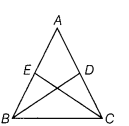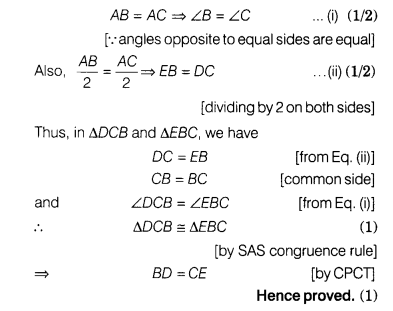# In the given figure, ABC is an isosceles triangle with AB = AC. BD and CE are two medians

In the given figure, ABC is an isosceles triangle with AB = AC. BD and CE are two medians of the triangle.

Prove that BD = CE.Given ABC is an isosceles triangle with AB = AC.
BD and CE are two medians.
To prove BD =CE
Proof In ∆ABC,